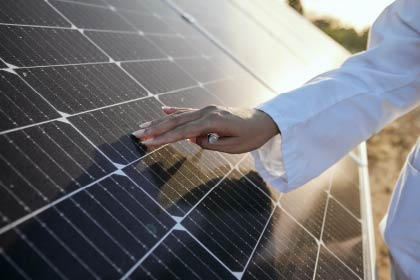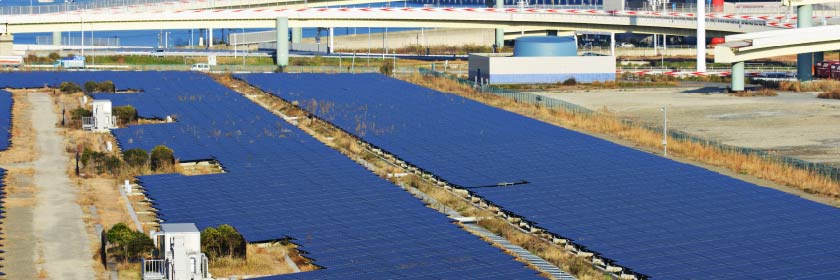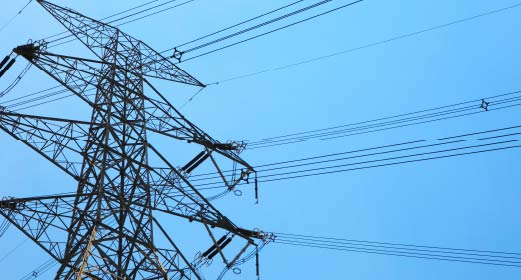# What is the difference between Power and Energy?

In physics and engineering, two of the most crucial ideas are power and energy. These words are frequently used in a number of disciplines, such as energy generation, electronics, and mechanics. While these two names can initially appear to be equivalent, there is a key distinction between them. While power describes the speed at which energy is transported or used up, energy relates to the ability to perform labor. This distinction must be understood in order for engineers, scientists, and technicians to create and improve systems that effectively utilize energy. Professionals who are aware of the differences between power and energy can better manage energy usage, maximize energy production, and make wise choices in real-world situations. Therefore, for anyone interested in engineering or physics, understanding these two ideas is fundamental because they are so important to our contemporary environment.

## Definition of Power

Power can be defined as the quantity of energy generated or consumed per unit of time, or the rate at which work is completed. The watt (W), a unit of power, is used to quantify the rate of energy transfer. Its definition is the amount of energy used or created per second. Power is typically measured properly using power meters, such as wattmeters or power analyzers. Power is a fundamental notion in the field of electrical engineering that is used to describe the pace of energy generation or consumption.

## Definition of Energy

In physics and engineering, the idea of energy describes a system’s ability to carry out work, including moving or heating objects. It can be assessed using specialist tools like calorimeters or energy meters and is measured in units of joules (J). For disciplines like engineering and physics, where it is utilized to define the capacity to do labor or complete tasks, understanding the idea of energy is essential. Energy is also a key factor in the production of energy, where limiting waste and improving performance depend on the efficient use of energy.Power can be defined as the quantity of energy generated or consumed per unit of time, or the rate at which work is completed.

## Differences between Power and Energy

Power and energy differ most significantly in how they are defined. Energy is the ability to perform work or complete tasks, whereas power is a measure of the rate at which energy is delivered. In other words, energy measures the entire amount of work that may be done, whereas power gauges how quickly energy is used up or moved. To put it another way, power is the pace of energy consumption, whereas energy is the total amount of energy utilized. This distinction is crucial in a variety of disciplines, including engineering and physics, where precisely measuring and applying these ideas is key for attaining the best results.

## Applications of Power and Energy

In physics and engineering, the idea of energy describes a system’s ability to carry out work, including moving or heating objects. It can be assessed using specialist tools like calorimeters or energy meters and is measured in units of joules (J). For disciplines like engineering and physics, where it is utilized to define the capacity to do labor or complete tasks, understanding the idea of energy is essential. Energy is also a key factor in the production of energy, where limiting waste and improving performance depend on the efficient use of energy.## Power

Uses of Power: Applications of power range from the household to the industrial, with examples including electric appliances, engines, and machinery. The output of engines and the energy consumption of electronic devices are two common examples of applications.

Importance of Power in Various Fields: Having a firm grasp on power is crucial for maximizing the efficiency of gadgets, motors, and machinery. Power is used to calculate the work done by a machine in mechanics and to measure the efficiency of power transmission in electrical engineering.

## Energy

Uses of Energy: Heating, cooling, moving around, and shining a light all necessitate energy. Electricity and transportation are both powered by fossil fuels, but renewable energy sources like solar and wind are gaining popularity.

Importance of Energy in Various Fields: To create and use effective energy sources, one must have a thorough understanding of energy. In the energy industry, the term “energy” refers to the total amount of energy produced, but in mechanics, it refers to the capacity to perform labor.### Conclusion:

To conclude, the distinction between power and energy is crucial in understanding how these concepts are applied in different fields. Even though power and energy are related concepts, they have distinct meanings that set them apart. Power describes the rate at which energy is transferred, while energy refers to the ability to perform work. Correctly applying these concepts in fields like electrical engineering, mechanics, and energy production requires an understanding of the differences between them. Thus, comprehending the relationship between power and energy is fundamental for optimizing energy use, improving the performance of devices and machines, and developing efficient energy sources. As such, understanding the distinction between power and energy is vital for anyone working in these fields.

### Get started with solar energy today

If you are looking to start your transition to solar energy, we are here to help. Our website also has a free online estimate tool that generates a personalized report for you from a simplified questionnaire. That report will contain the potential savings you can net, and the cost of a solar energy system installed on your property.